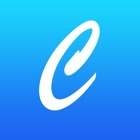• 搜索类型

所有分类iPhone 应用程序

所有分类iPhone 游戏# C++编程

0 0

## 截屏

描述

C++ 是一种中级语言，它是由 Bjarne Stroustrup 于 1979 年在贝尔实验室开始设计开发的。C++ 进一步扩充和完善了 C 语言，是一种面向对象的程序设计语言。C++ 可运行于多种平台上。
本教程通过通俗易懂的语言来讲解 C++ 编程语言。
教程包含以下章节
基础教程
*C++ 简介
*C++ 环境设置
*C++ 基本语法
*C++ 注释
*C++ 数据类型
*C++ 变量类型
*C++ 变量作用域
*C++ 常量
*C++ 修饰符类型
*C++ 存储类
*C++ 运算符
*C++ 循环
*C++ 判断
*C++ 函数
*C++ 数字
*C++ 数组
*C++ 字符串
*C++ 指针
*C++ 引用
*C++ 日期 & 时间
*C++ 基本的输入输出
*C++ 数据结构

C++ 面向对象
*C++ 类 & 对象
*C++ 继承
*C++ 重载运算符和重载函数
*C++ 多态
*C++ 数据抽象
*C++ 数据封装
*C++ 接口（抽象类）

C++ 高级教程
*C++ 文件和流
*C++ 异常处理
*C++ 动态内存
*C++ 命名空间
*C++ 模板
*C++ 预处理器
*C++ 信号处理
*C++ 多线程
*C++ Web 编程

C++ 资源库
*C++ STL 教程
*C++ 标准库
*C++ 有用的资源